# Lesson 8 Graphical Differentiation Calculus Santowski 1102022 Calculus

• Slides: 25Lesson 8 - Graphical Differentiation Calculus - Santowski 1/10/2022 Calculus - Santowski 1Lesson Objectives • 1. Given the equation of a function, graph it and then make conjectures about the relationship between the derivative function and the original function • 2. From a function, sketch its derivative • 3. From a derivative, graph an original function 1/10/2022 Calculus - Santowski 2Fast Five • 1. Find f(x) if d/dx f(x) = -x 2 + 2 x • 2. Sketch a graph whose first derivative is always negative • 3. Graph the derivative of the function • 4. If the graph represented the derivative, sketch the original function 1/10/2022 Calculus - Santowski 3(A) Important Terms • • turning point: maximum: minimum: increase: decrease: “concave up” “concave down” 1/10/2022 Calculus - Santowski 4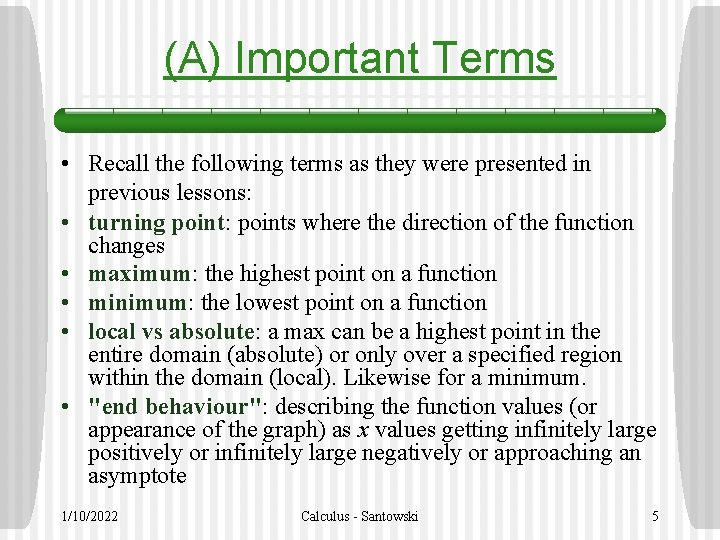(A) Important Terms • Recall the following terms as they were presented in previous lessons: • turning point: points where the direction of the function changes • maximum: the highest point on a function • minimum: the lowest point on a function • local vs absolute: a max can be a highest point in the entire domain (absolute) or only over a specified region within the domain (local). Likewise for a minimum. • "end behaviour": describing the function values (or appearance of the graph) as x values getting infinitely large positively or infinitely large negatively or approaching an asymptote 1/10/2022 Calculus - Santowski 5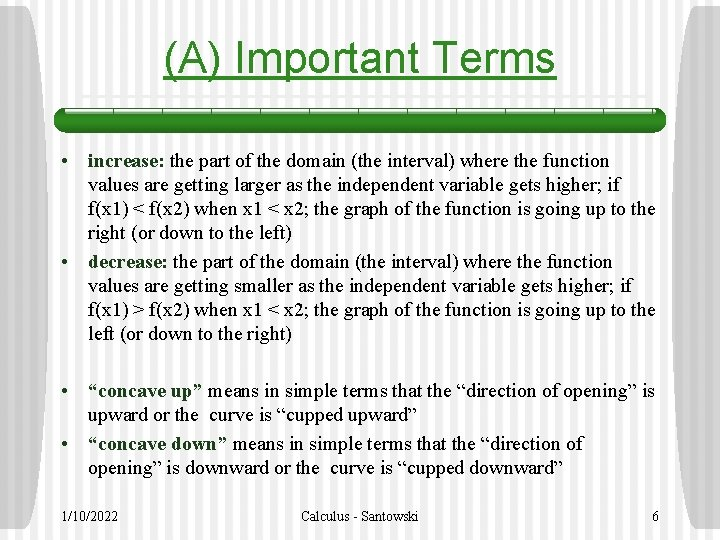(A) Important Terms • increase: the part of the domain (the interval) where the function values are getting larger as the independent variable gets higher; if f(x 1) < f(x 2) when x 1 < x 2; the graph of the function is going up to the right (or down to the left) • decrease: the part of the domain (the interval) where the function values are getting smaller as the independent variable gets higher; if f(x 1) > f(x 2) when x 1 < x 2; the graph of the function is going up to the left (or down to the right) • “concave up” means in simple terms that the “direction of opening” is upward or the curve is “cupped upward” • “concave down” means in simple terms that the “direction of opening” is downward or the curve is “cupped downward” 1/10/2022 Calculus - Santowski 6(A) Important Terms 1/10/2022 Calculus - Santowski 7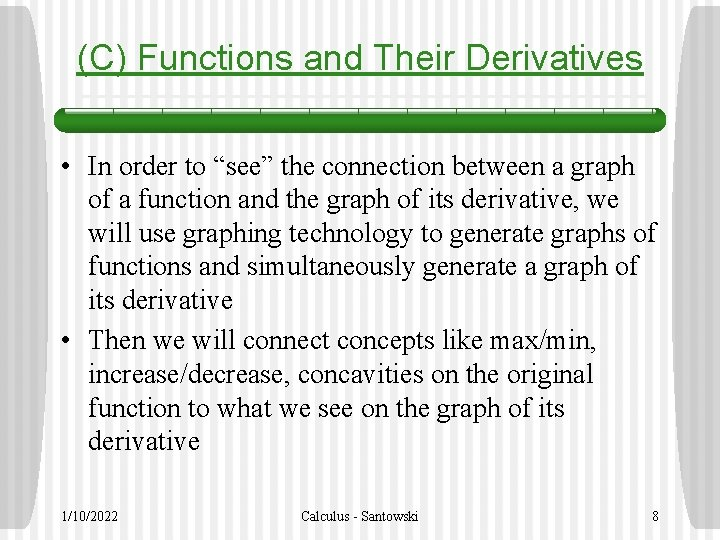(C) Functions and Their Derivatives • In order to “see” the connection between a graph of a function and the graph of its derivative, we will use graphing technology to generate graphs of functions and simultaneously generate a graph of its derivative • Then we will connect concepts like max/min, increase/decrease, concavities on the original function to what we see on the graph of its derivative 1/10/2022 Calculus - Santowski 8(D) Example #1 1/10/2022 Calculus - Santowski 9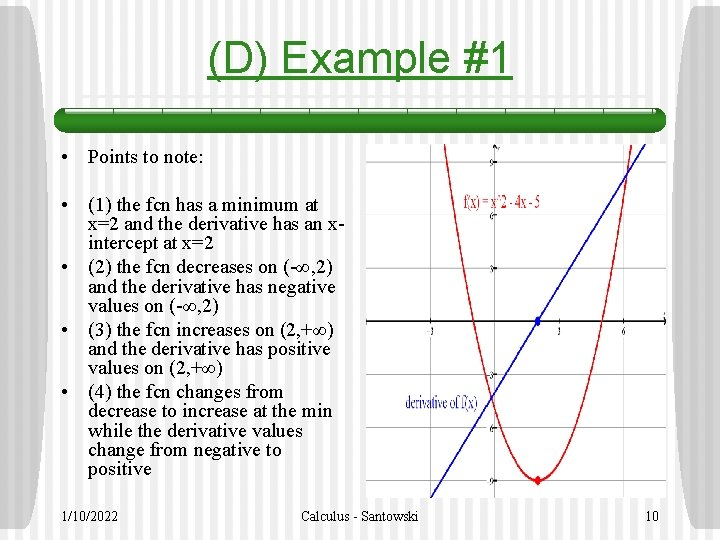(D) Example #1 • Points to note: • (1) the fcn has a minimum at x=2 and the derivative has an xintercept at x=2 • (2) the fcn decreases on (-∞, 2) and the derivative has negative values on (-∞, 2) • (3) the fcn increases on (2, +∞) and the derivative has positive values on (2, +∞) • (4) the fcn changes from decrease to increase at the min while the derivative values change from negative to positive 1/10/2022 Calculus - Santowski 10(D) Example #1 • Points to note: • (5) the function is concave up and the derivative fcn is an increasing fcn • (6) The second derivative of f(x) is positive 1/10/2022 Calculus - Santowski 11(E) Example #2 1/10/2022 Calculus - Santowski 12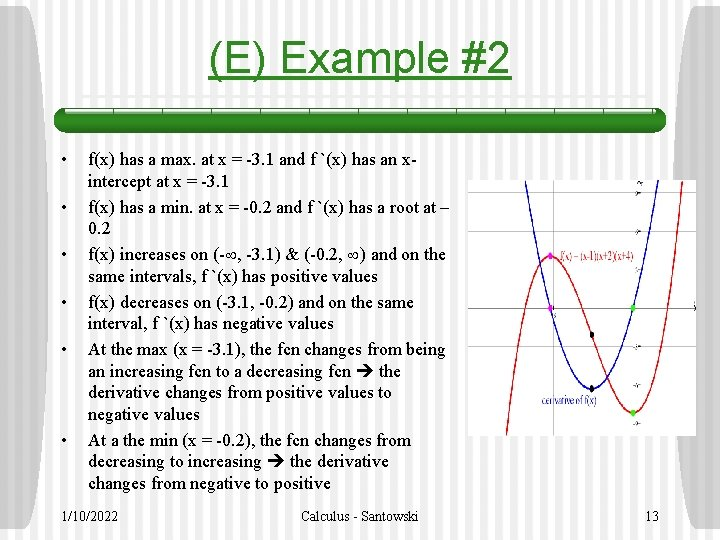(E) Example #2 • • • f(x) has a max. at x = -3. 1 and f `(x) has an xintercept at x = -3. 1 f(x) has a min. at x = -0. 2 and f `(x) has a root at – 0. 2 f(x) increases on (- , -3. 1) & (-0. 2, ) and on the same intervals, f `(x) has positive values f(x) decreases on (-3. 1, -0. 2) and on the same interval, f `(x) has negative values At the max (x = -3. 1), the fcn changes from being an increasing fcn to a decreasing fcn the derivative changes from positive values to negative values At a the min (x = -0. 2), the fcn changes from decreasing to increasing the derivative changes from negative to positive 1/10/2022 Calculus - Santowski 13(E) Example #2 • • • At the max (x = -3. 1), the fcn changes from being an increasing fcn to a decreasing fcn the derivative changes from positive values to negative values At a the min (x = -0. 2), the fcn changes from decreasing to increasing the derivative changes from negative to positive f(x) is concave down on (- , -1. 67) while f `(x) decreases on (- , -1. 67) f(x) is concave up on (-1. 67, ) while f `(x) increases on (-1. 67, ) The concavity of f(x) changes from CD to CU at x = -1. 67, while the derivative has a min. at x = 1. 67 1/10/2022 Calculus - Santowski 14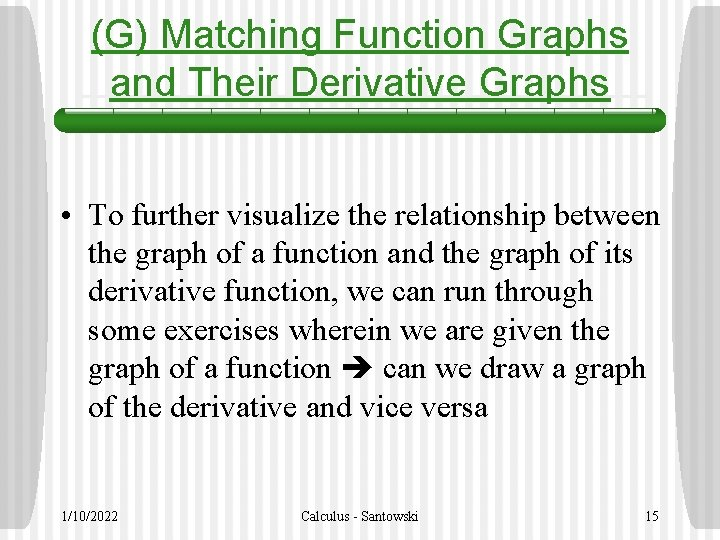(G) Matching Function Graphs and Their Derivative Graphs • To further visualize the relationship between the graph of a function and the graph of its derivative function, we can run through some exercises wherein we are given the graph of a function can we draw a graph of the derivative and vice versa 1/10/2022 Calculus - Santowski 15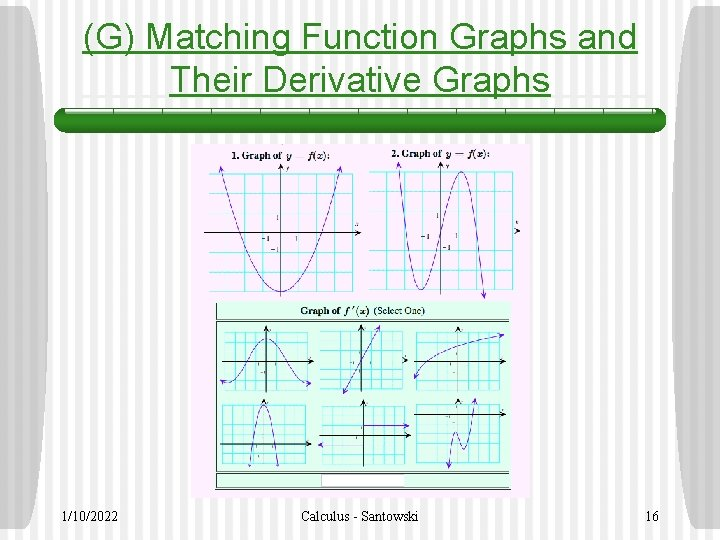(G) Matching Function Graphs and Their Derivative Graphs 1/10/2022 Calculus - Santowski 16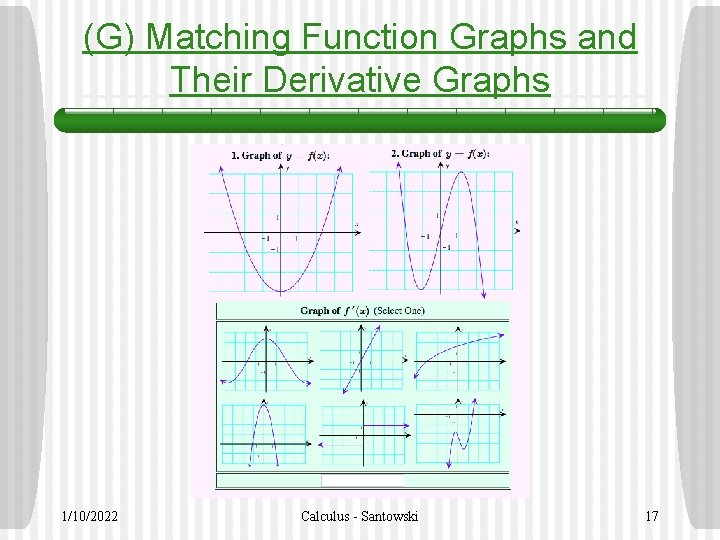(G) Matching Function Graphs and Their Derivative Graphs 1/10/2022 Calculus - Santowski 17(G) Matching Function Graphs and Their Derivative Graphs 1/10/2022 Calculus - Santowski 18(G) Matching Function Graphs and Their Derivative Graphs 1/10/2022 Calculus - Santowski 19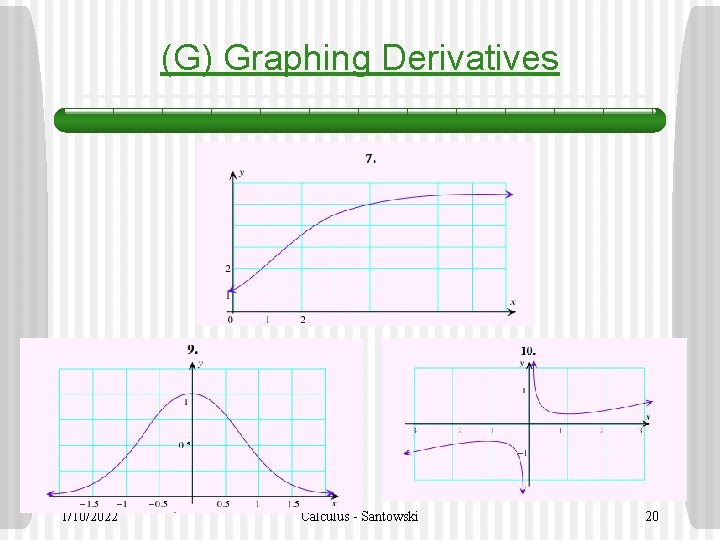(G) Graphing Derivatives 1/10/2022 Calculus - Santowski 20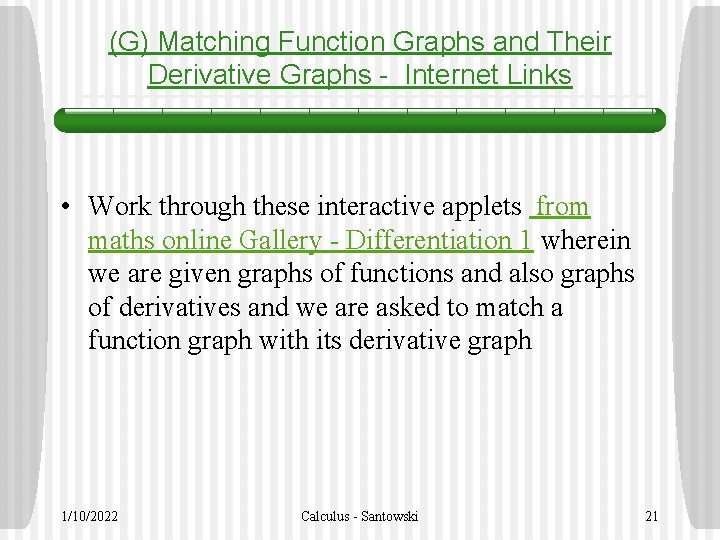(G) Matching Function Graphs and Their Derivative Graphs - Internet Links • Work through these interactive applets from maths online Gallery - Differentiation 1 wherein we are given graphs of functions and also graphs of derivatives and we are asked to match a function graph with its derivative graph 1/10/2022 Calculus - Santowski 21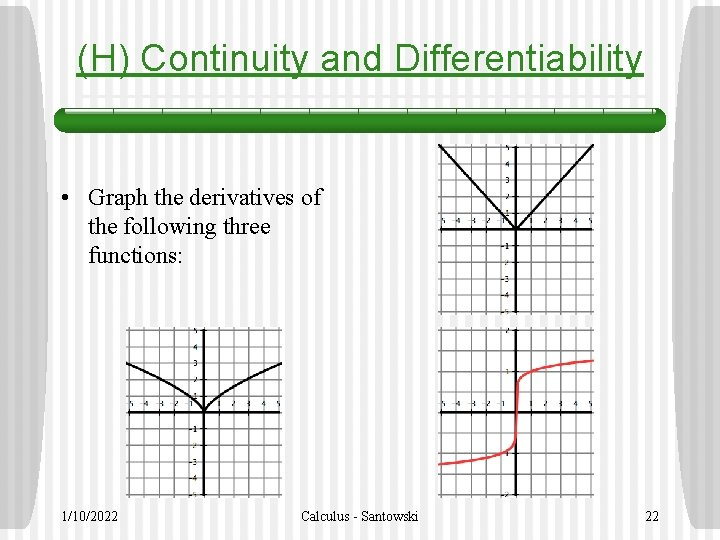(H) Continuity and Differentiability • Graph the derivatives of the following three functions: 1/10/2022 Calculus - Santowski 22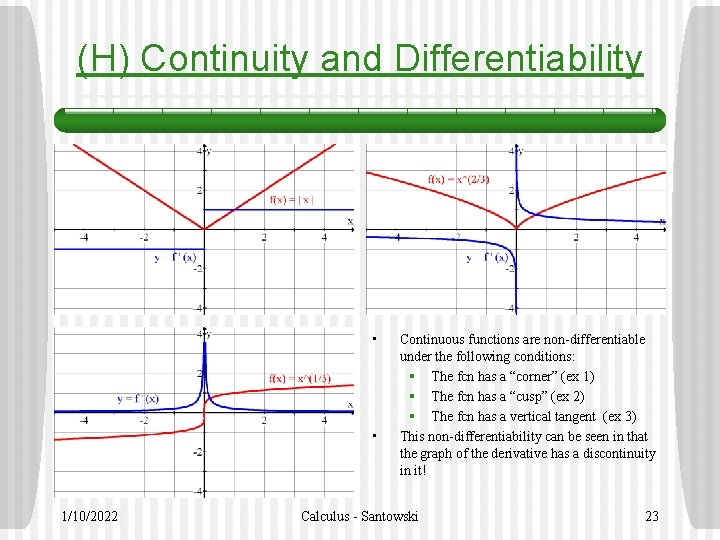(H) Continuity and Differentiability • • 1/10/2022 Continuous functions are non-differentiable under the following conditions: § The fcn has a “corner” (ex 1) § The fcn has a “cusp” (ex 2) § The fcn has a vertical tangent (ex 3) This non-differentiability can be seen in that the graph of the derivative has a discontinuity in it! Calculus - Santowski 23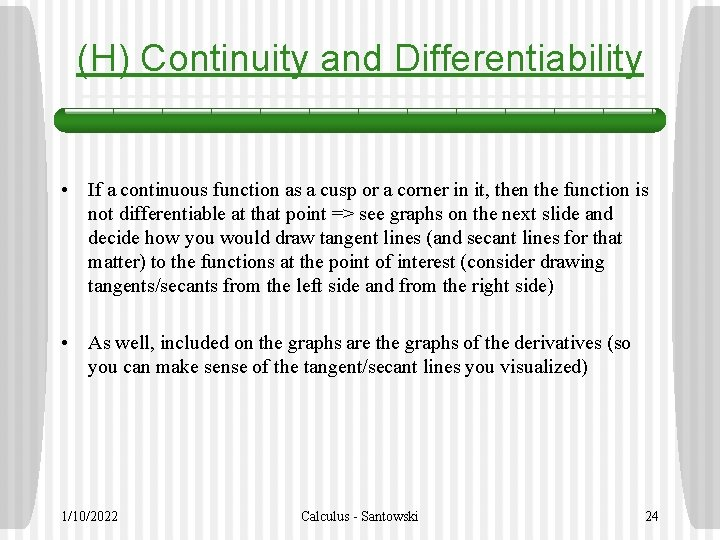(H) Continuity and Differentiability • If a continuous function as a cusp or a corner in it, then the function is not differentiable at that point => see graphs on the next slide and decide how you would draw tangent lines (and secant lines for that matter) to the functions at the point of interest (consider drawing tangents/secants from the left side and from the right side) • As well, included on the graphs are the graphs of the derivatives (so you can make sense of the tangent/secant lines you visualized) 1/10/2022 Calculus - Santowski 24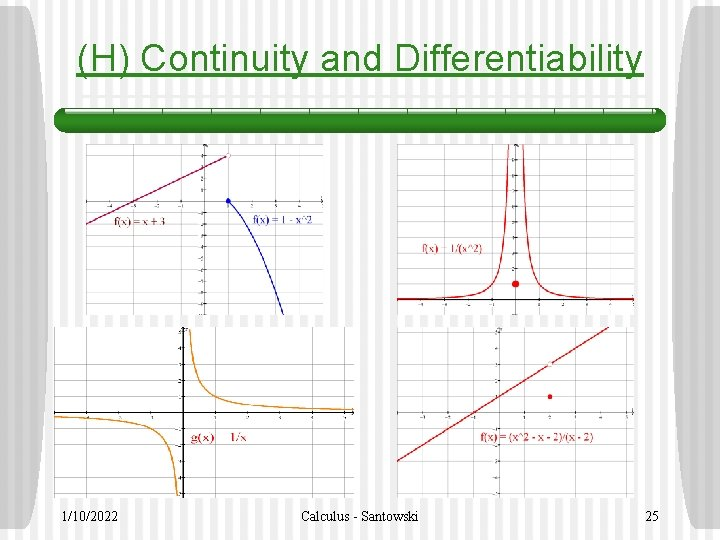(H) Continuity and Differentiability 1/10/2022 Calculus - Santowski 25